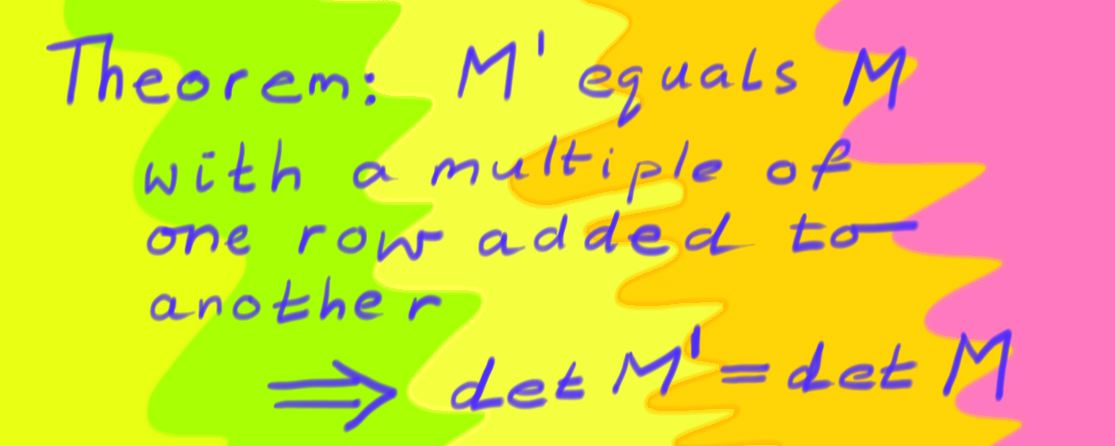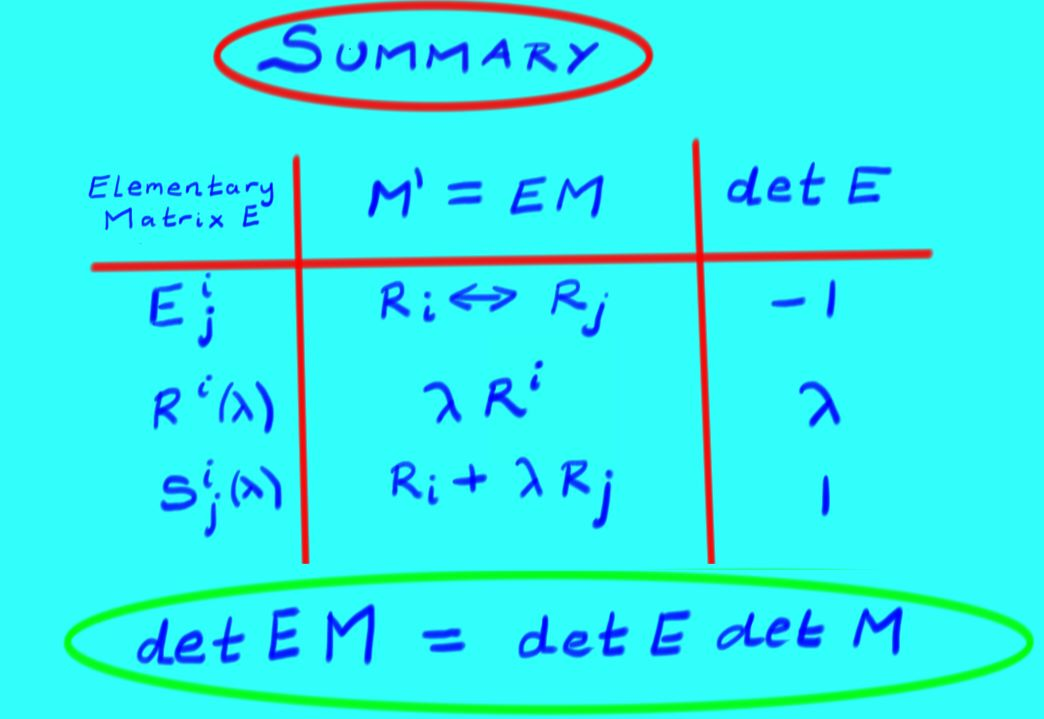$$\newcommand{\id}{\mathrm{id}}$$ $$\newcommand{\Span}{\mathrm{span}}$$ $$\newcommand{\kernel}{\mathrm{null}\,}$$ $$\newcommand{\range}{\mathrm{range}\,}$$ $$\newcommand{\RealPart}{\mathrm{Re}}$$ $$\newcommand{\ImaginaryPart}{\mathrm{Im}}$$ $$\newcommand{\Argument}{\mathrm{Arg}}$$ $$\newcommand{\norm}{\| #1 \|}$$ $$\newcommand{\inner}{\langle #1, #2 \rangle}$$ $$\newcommand{\Span}{\mathrm{span}}$$

8.2: Elementary Matrices and Determinants

$$\newcommand{\vecs}{\overset { \rightharpoonup} {\mathbf{#1}} }$$ $$\newcommand{\vecd}{\overset{-\!-\!\rightharpoonup}{\vphantom{a}\smash {#1}}}$$$$\newcommand{\id}{\mathrm{id}}$$ $$\newcommand{\Span}{\mathrm{span}}$$ $$\newcommand{\kernel}{\mathrm{null}\,}$$ $$\newcommand{\range}{\mathrm{range}\,}$$ $$\newcommand{\RealPart}{\mathrm{Re}}$$ $$\newcommand{\ImaginaryPart}{\mathrm{Im}}$$ $$\newcommand{\Argument}{\mathrm{Arg}}$$ $$\newcommand{\norm}{\| #1 \|}$$ $$\newcommand{\inner}{\langle #1, #2 \rangle}$$ $$\newcommand{\Span}{\mathrm{span}}$$ $$\newcommand{\id}{\mathrm{id}}$$ $$\newcommand{\Span}{\mathrm{span}}$$ $$\newcommand{\kernel}{\mathrm{null}\,}$$ $$\newcommand{\range}{\mathrm{range}\,}$$ $$\newcommand{\RealPart}{\mathrm{Re}}$$ $$\newcommand{\ImaginaryPart}{\mathrm{Im}}$$ $$\newcommand{\Argument}{\mathrm{Arg}}$$ $$\newcommand{\norm}{\| #1 \|}$$ $$\newcommand{\inner}{\langle #1, #2 \rangle}$$ $$\newcommand{\Span}{\mathrm{span}}$$

In chapter 2 we found the elementary matrices that perform the Gaussian row operations. In other words, for any matrix $$M$$, and a matrix $$M'$$ equal to $$M$$ after a row operation, multiplying by an elementary matrix $$E$$ gave $$M'=EM$$. We now examine what the elementary matrices to do determinants.

8.2.1 Row Swap

Our first elementary matrix multiplies a matrix $$M$$ by swapping rows $$i$$ and $$j$$. Explicitly: let $$R^{1}$$ through $$R^{n}$$ denote the rows of $$M$$, and let $$M'$$ be the matrix $$M$$ with rows $$i$$ and $$j$$ swapped. Then $$M$$ and $$M'$$ can be regarded as a block matrices (where the blocks are rows):

$M=\begin{pmatrix}\vdots \\ R^{i} \\ \vdots \\ R^{j} \\ \vdots\end{pmatrix} \textit{ and } M'=\begin{pmatrix}\vdots \\ R^{j} \\ \vdots \\ R^{i} \\ \vdots\end{pmatrix}.$

Then notice that:

$M'=\begin{pmatrix} \vdots \\ R^{j} \\ \vdots \\ R^{i} \\ \vdots\\ \\ \end{pmatrix}= \begin{pmatrix} 1 & & & & & & \\ & \ddots & & & & & \\ & & 0 & & 1 & & \\ & & & \ddots & & & \\ & & 1 & & 0 & & \\ & & & & & \ddots & \\ & & & & & & 1 \\ \end{pmatrix} \begin{pmatrix} \vdots \\ R^{i} \\ \vdots \\ R^{j} \\ \vdots \\ \\ \end{pmatrix}$

The matrix

$\begin{pmatrix} 1 & & & & & & \\ & \ddots & & & & & \\ & & 0 & & 1 & & \\ & & & \ddots & & & \\ & & 1 & & 0 & & \\ & & & & & \ddots & \\ & & & & & & 1 \\ \end{pmatrix}=:E^{i}_{j}$

is just the identity matrix with rows $$i$$ and $$j$$ swapped. The matrix $$E^{i}_{j}$$ is an $$\textit{elementary matrix}$$ and

$M'=E^{i}_{j}M\, .$

Because $$\det I=1$$ and swapping a pair of rows changes the sign of the determinant, we have found that

$\det E^{i}_{j} = -1\, .$

Now we know that swapping a pair of rows flips the sign of the determinant so $$\det M'=-det M$$. But $$\det E_{j}^{i}=-1$$ and $$M'=E^{i}_{j} M$$ so

$\det E^{i}_{j} M = \det E^{i}_{j} \, \det M\, .$

This result hints at a general rule for determinants of products of matrices.

8.2.2 Scalar Multiply

The next row operation is multiplying a row by a scalar. Consider $$M=\begin{pmatrix}R^{1} \\ \vdots \\ R^{n} \end{pmatrix}\, ,$$ where $$R^{i}$$ are row vectors. Let $$R^{i}(\lambda)$$ be the identity matrix, with the $$i$$th diagonal entry replaced by $$\lambda$$, not to be confused with the row vectors. $$\textit{i.e.}$$,

$R^{i}(\lambda)= \begin{pmatrix} 1 & & & & \\ & \ddots & & & \\ & & \lambda & & \\ & & & \ddots & \\ & & & & 1 \\ \end{pmatrix} \, .$

Then:

$M'=R^{i}(\lambda)M=\begin{pmatrix}R^{1} \\ \vdots \\ \lambda R^{i} \\ \vdots \\ R^{n} \end{pmatrix}\, ,$

equals $$M$$ with one row multiplied by $$\lambda$$.

What effect does multiplication by the elementary matrix $$R^{i}(\lambda)$$ have on the determinant?

\begin{eqnarray*}
\det M' & = & \sum_{\sigma} \textit{sgn}(\sigma) m^{1}_{\sigma(1)}\cdots \lambda m^{i}_{\sigma(i)} \cdots m^{n}_{\sigma(n)} \\
& = & \lambda \sum_{\sigma} \textit{sgn}(\sigma) m^{1}_{\sigma(1)}\cdots m^{i}_{\sigma(i)} \cdots m^{n}_{\sigma(n)} \\
& = & \lambda \det M
\end{eqnarray*}

Thus, multiplying a row by $$\lambda$$ multiplies the determinant by $$\lambda$$. $$\textit{i.e.,}$$ $$\det R^{i}(\lambda) M = \lambda \det M\, .\]Since $$R^{i}(\lambda)$$ is just the identity matrix with a single row multiplied by $$\lambda$$, then by the above rule, the determinant of $$R^{i}(\lambda)$$ is $$\lambda$$. Thus: $\det R^{i}(\lambda) = \det \begin{pmatrix} 1 & & & & \\ & \ddots & & & \\ & & \lambda & & \\ & & & \ddots & \\ & & & & 1 \\ \end{pmatrix} = \lambda\, ,$ and once again we have a product of determinants formula:$$
\det R^{i}(\lambda) M = \det R^{i}(\lambda) \det M
\]

The final row operation is adding $$\mu R^{j}$$ to $$R^{i}$$. This is done with the elementary matrix $$S^{i}_{j}(\mu)$$, which is an identity matrix but with an additional $$\mu$$ in the $$i,j$$ position:

$S^{i}_{j}(\mu) = \begin{pmatrix} 1 & & & & & & \\ & \ddots & & & & & \\ & & 1 & & \mu & & \\ & & & \ddots & & & \\ & & & & 1 & & \\ & & & & & \ddots & \\ & & & & & & 1 \\ \end{pmatrix}\, .$
Then multiplying $$M$$ by $$S^{i}_{j}(\mu)$$ performs a row addition:

$\begin{pmatrix} 1 & & & & & & \\ & \ddots & & & & & \\ & & 1 & & \mu & & \\ & & & \ddots & & & \\ & & & & 1 & & \\ & & & & & \ddots & \\ & & & & & & 1 \\ \end{pmatrix}\begin{pmatrix}\\ \vdots \\ R^{i} \\ \vdots \\ R^{j} \\ \vdots\\ \\\end{pmatrix} = \begin{pmatrix}\\ \vdots \\ R^{i} +\mu R^{j} \\ \vdots \\ R^{j} \\ \vdots\\ \\ \end{pmatrix}\, .$
What is the effect of multiplying by $$S^{i}_{j}(\mu)$$ on the determinant? Let $$M'=S^{i}_{j}(\mu)M$$, and let $$M''$$ be the matrix $$M$$ but with $$R^{i}$$ replaced by $$R^{j}$$. Then

\begin{eqnarray*}
\det M' & = & \sum_{\sigma} \textit{sgn}(\sigma) m^{1}_{\sigma(1)}\cdots (m^{i}_{\sigma(i)}+ \mu m^{j}_{\sigma(j)}) \cdots m^{n}_{\sigma(n)} \\
& = & \sum_{\sigma} \textit{sgn}(\sigma) m^{1}_{\sigma(1)}\cdots m^{i}_{\sigma(i)} \cdots m^{n}_{\sigma(n)} \\
& & \qquad + \sum_{\sigma} \textit{sgn}(\sigma) m^{1}_{\sigma(1)}\cdots \mu m^{j}_{\sigma(j)} \cdots m^{j}_{\sigma(j)} \cdots m^{n}_{\sigma(n)} \\
& = & \det M + \mu \det M''
\end{eqnarray*}
Since $$M''$$ has two identical rows, its determinant is $$0$$ so
$$\det M' = \det M,$$
when $$M'$$ is obtained from $$M$$ by adding $$\mu$$ times row $$j$$ to row $$i$$.

We also have learnt that
$$\det S^{i}_{j}(\mu)M = \det M\, .$$
Notice that if $$M$$ is the identity matrix, then we have $$\det S^{i}_{j}(\mu) = \det (S^{i}_{j}(\mu)I) = \det I = 1\, .\]8.2.4 Determinant of Products In summary, the elementary matrices for each of the row operations obey $\begin{array}{cccc} E^{i}_{j} &=& I \textit{ with rows i,j swapped;} &\det E^{i}_{j}=-1 \\ R^{i}(\lambda) &=& I \textit{ with $$\lambda$$ in position i,i;} &\det R^{i}(\lambda)=\lambda \\S^{i}_{j}(\mu) &=& I \textit{ with \mu in position i,j;} &\det S^{i}_{j}(\mu)=1 \\ \end{array}$ Moreover we found a useful formula for determinants of products: Theorem If $$E$$ is $$\textit{any}$$ of the elementary matrices $$E^{i}_{j}, R^{i}(\lambda), S^{i}_{j}(\mu)$$, then $$\det(EM)=\det E \det M$$.We have seen that any matrix $$M$$ can be put into reduced row echelon form via a sequence of row operations, and we have seen that any row operation can be achieved via left matrix multiplication by an elementary matrix. Suppose that RREF$$(M)$$ is the reduced row echelon form of $$M$$. Then$$RREF(M)=E_{1}E_{2}\cdots E_{k}M\, ,$$where each $$E_{i}$$ is an elementary matrix. We know how to compute determinants of elementary matrices and products thereof, so we ask:$$\textit{What is the determinant of a square matrix in reduced row echelon form? }$$The answer has two cases: 1. If $$M$$ is not invertible, then some row of $$RREF(M)$$ contains only zeros. Then we can multiply the zero row by any constant $$\lambda$$ without changing $$M$$; by our previous observation, this scales the determinant of $$M$$ by $$\lambda$$. Thus, if $$M$$ is not invertible, $$\det RREF(M)=\lambda \det RREF(M)$$, and so $$\det RREF(M)=0$$. 2. Otherwise, every row of $$RREF(M)$$ has a pivot on the diagonal; since $$M$$ is square, this means that $$RREF(M)$$ is the identity matrix. So if $$M$$ is invertible, $$\det RREF(M)=1$$. Notice that because $$\det RREF(M) = \det (E_{1}E_{2}\cdots E_{k}M)$$, by the theorem above,$$\det RREF(M)=\det (E_{1}) \cdots \det (E_{k}) \det M\, .$$Since each $$E_{i}$$ has non-zero determinant, then $$\det RREF(M)=0$$ if and only if $$\det M=0$$. This establishes an important theorem: Theorem $$\textit{For any square matrix M, \det M\neq 0 if and only if M is invertible.}$$ Since we know the determinants of the elementary matrices, we can immediately obtain the following:Corollary Any elementary matrix $$E^{i}_{j}, R^i(\lambda), S^{i}_{j}(\mu)$$ is invertible, except for $$R^{i}(0)$$. In fact, the inverse of an elementary matrix is another elementary matrix. To obtain one last important result, suppose that $$M$$ and $$N$$ are square $$n\times n$$ matrices, with reduced row echelon forms such that, for elementary matrices $$E_{i}$$ and $$F_{i}$$,$$M=E_{1}E_{2}\cdots E_{k} \, RREF(M)\, ,$$and$$N=F_{1}F_{2}\cdots F_{l} \, RREF(N)\, . If $$RREF(M)$$ is the identity matrix ($$\textit{i.e.}$$, $$M$$ is invertible), then:

\begin{eqnarray*}
\det (MN) & = & \det (E_{1}E_{2}\cdots E_{k}\, RREF(M) F_{1}F_{2}\cdots F_{l} \, RREF(N) )\\
& = & \det (E_{1}E_{2}\cdots E_{k} I F_{1}F_{2}\cdots F_{l}\, RREF(N) )\\
& = & \det (E_{1}) \cdots \det(E_{k})\det(I)\det(F_{1})\cdots\det(F_{l})\det RREF(N)\\
& = & \det(M)\det(N)
\end{eqnarray*}
Otherwise, $$M$$ is not invertible, and $$\det M=0=\det RREF(M)$$. Then there exists a row of zeros in $$RREF(M)$$, so $$R^{n}(\lambda) RREF(M)=RREF(M)$$ $$\textit{for any \lambda}$$. Then:\begin{eqnarray*}\det (MN) & = & \det (E_{1}E_{2}\cdots E_{k}
\, RREF(M) N )\\
& = & \det (E_{1}E_{2}\cdots E_{k}
\, RREF(M) N )\\
& = & \det (E_{1}) \cdots \det(E_{k})\det(
RREF(M)N)\\
& = & \det (E_{1}) \cdots \det(E_{k})\det( R^{n}(\lambda)
\, RREF(M)N)\\
& = & \det (E_{1}) \cdots \det(E_{k})\lambda \det(
RREF(M)N)\\
& = & \lambda \det (MN)
\end{eqnarray*}
Which implies that $$\det (MN)=0=\det M \det N$$.

Thus we have shown that for $$\textit{any}$$ matrices $$M$$ and $$N$$,
$\det (MN) = \det M \det N$
This result is $$\textit{extremely important}$$; do not forget it!